Main Content

# na_0002: Appropriate usage of basic logical and numerical operations

## Sub ID Recommendations

• NA-MAAB — a, b

• JMAAB — a, b

All

## Rule

### Sub ID a

Logical signals shall not connect to blocks that operate on numerical signals.

Custom Parameter

Blocks receiving numerical signals

Example — Correct

Numerical values are compared to determine if they are equal.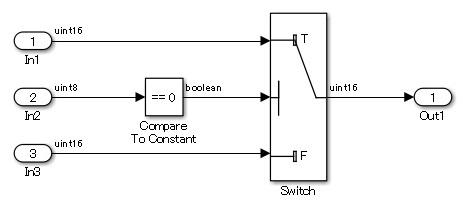Example — Incorrect

A logical output is connected directly to the input of blocks that process numerical inputs.A logical signal is compared with a numerical value.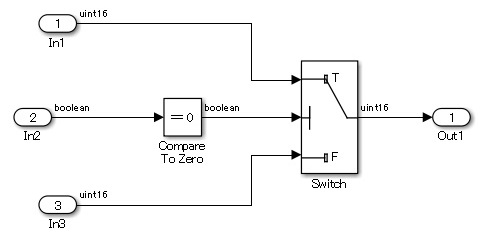### Sub ID b

Numerical signals shall not connect to blocks that operate on logical signals.

Custom Parameter

Blocks receiving logical signals

Example — Correct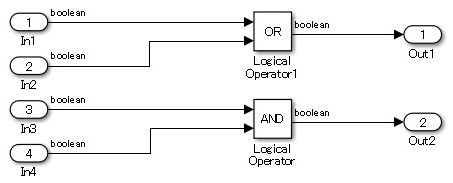Logical signal is inverted by using a logical operation.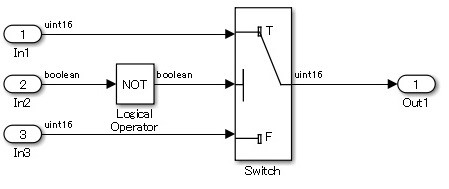Logical signal is evaluated by using a logical operation.Example — Incorrect

Logical signal is inverted by using a logical operation.

A block that is used to perform logical operations is being used to perform numerical operations. A numerical output is connected to the input of blocks that process logical inputs.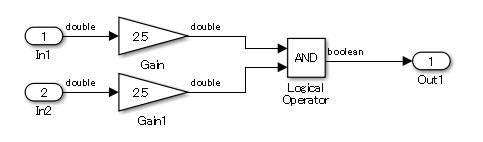A block that is used to perform numerical operations is being used to perform logical operations. Inputs other than logical values can be provided to the block. However, the Enable port block can receive only logical signals that have `On`/`Off`. The Product block performs logical operations when it connects the numerical operations result to a block that receives the logical value Enable port.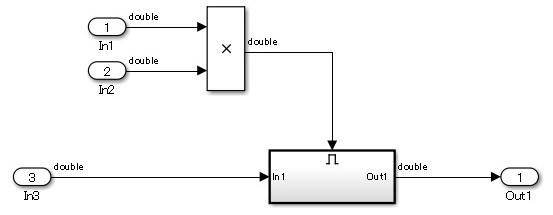## Rationale

Sub IDs a, b:

• When numerical and logical values are treated the same, the original intention becomes unclear and the next operation in the model can be incorrectly interpreted, further compounding the error.

## Verification

Model Advisor check: Check fundamental logical and numerical operations (Simulink Check)

R2020a

## See Also

Introduced in R2020a

## Support

#### Model-Based Design for Embedded Control Systems

Download white paper